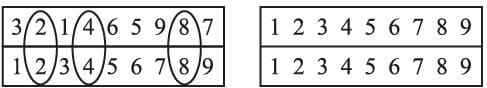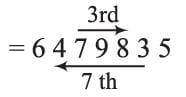Olympiad Test: Number, Ranking & Time Sequence

# Olympiad Test: Number, Ranking & Time Sequence

Test Description

## 15 Questions MCQ Test Science Olympiad Class 9 | Olympiad Test: Number, Ranking & Time Sequence

Olympiad Test: Number, Ranking & Time Sequence for Class 9 2022 is part of Science Olympiad Class 9 preparation. The Olympiad Test: Number, Ranking & Time Sequence questions and answers have been prepared according to the Class 9 exam syllabus.The Olympiad Test: Number, Ranking & Time Sequence MCQs are made for Class 9 2022 Exam. Find important definitions, questions, notes, meanings, examples, exercises, MCQs and online tests for Olympiad Test: Number, Ranking & Time Sequence below.
Solutions of Olympiad Test: Number, Ranking & Time Sequence questions in English are available as part of our Science Olympiad Class 9 for Class 9 & Olympiad Test: Number, Ranking & Time Sequence solutions in Hindi for Science Olympiad Class 9 course. Download more important topics, notes, lectures and mock test series for Class 9 Exam by signing up for free. Attempt Olympiad Test: Number, Ranking & Time Sequence | 15 questions in 15 minutes | Mock test for Class 9 preparation | Free important questions MCQ to study Science Olympiad Class 9 for Class 9 Exam | Download free PDF with solutions
 1 Crore+ students have signed up on EduRev. Have you?
Olympiad Test: Number, Ranking & Time Sequence - Question 1

### Raju is 5th from the left and Pankaj is 12th from the right end in a row of students. If Pankaj shifts three places towards Raju, his position is 10th from the left. How many students are there in the row?

Detailed Solution for Olympiad Test: Number, Ranking & Time Sequence - Question 1

When Pankaj shifts 3 places towards Raju his position is 15th from right and 10th from left.
∴ No. of students = 15 + 10 – 1 = 24 students.

Olympiad Test: Number, Ranking & Time Sequence - Question 2

### In a particular year, 1st November is Wednesday what day was 1st October in that year?

Detailed Solution for Olympiad Test: Number, Ranking & Time Sequence - Question 2

1st November is Wednesday.
31st October is Tuesday.
29th, 22th, 15th, 8th, 1st October was Sundays.

Olympiad Test: Number, Ranking & Time Sequence - Question 3

### Some students stand in a row. Manish’s position is 19th from both ends. How many students are there in that row?

Detailed Solution for Olympiad Test: Number, Ranking & Time Sequence - Question 3

Manish’s position is 19th from both ends
Total no. of students = 19 + 19 – 1
= 38 – 1
= 37.

Olympiad Test: Number, Ranking & Time Sequence - Question 4

Navin ranks 18th in a class of 49 students. What is his rank from the last?

Detailed Solution for Olympiad Test: Number, Ranking & Time Sequence - Question 4

Here, Number students behind the nitin in rank = 49 – 18 = 31
Navin’s rank from last = 32nd.

Olympiad Test: Number, Ranking & Time Sequence - Question 5

The position of how many digits in the number 321465987 will remain the same when the digits are arranged in ascending order?

Detailed Solution for Olympiad Test: Number, Ranking & Time Sequence - Question 5Olympiad Test: Number, Ranking & Time Sequence - Question 6

The position of the 1st and 5th digits in the number 83256479 are interchanged. Similarly, the position of the 2nd and 6th digits are interchanged. Which of the following is the third to the right of the 7th digit from the right end after the rearrangement?

Detailed Solution for Olympiad Test: Number, Ranking & Time Sequence - Question 6

The new number after rearrangement7th digit from right end = 4
3rd digit to the right of 4 = 8.

Olympiad Test: Number, Ranking & Time Sequence - Question 7

How many numbers amongst the number 9 to 81 are there which are divisible by 9 but not by 3?

Detailed Solution for Olympiad Test: Number, Ranking & Time Sequence - Question 7

There is no such number that is divisible by 9 but not by 3.

Olympiad Test: Number, Ranking & Time Sequence - Question 8

If Aman find that he is 12th from the right in a line of boys and 4th from the left. How many boys should be added to the line such that there are 28 boys in the line?

Detailed Solution for Olympiad Test: Number, Ranking & Time Sequence - Question 8

Number of boys to the right of Anish = 12 - 1 = 11 boys
Total number of boys in the row = position of Anish from left + number of boys to the right of Anish = 4 + 11 = 15
Therefore, the number of boys to be added to the line such that there are 28 boys in the line be x
Then,
15 + x = 28
x = 28 - 15
x = 13
Hence, 13 is the correct answer.

Olympiad Test: Number, Ranking & Time Sequence - Question 9

If the 25th of August in a year is Thursday, the number of Mondays in that month is

Detailed Solution for Olympiad Test: Number, Ranking & Time Sequence - Question 9

25th August is Thursday.
29th August is Monday.
Then 29th, 22th, 15th, 8th, and 1st days are Monday.
So, there are 5 Monday in that month.

Olympiad Test: Number, Ranking & Time Sequence - Question 10

Which two months in a year have the same calendar?

Detailed Solution for Olympiad Test: Number, Ranking & Time Sequence - Question 10

April and July have the same calendar.

Olympiad Test: Number, Ranking & Time Sequence - Question 11

Aman is 6th from the left end and Bimal is 10th from the right end in a row of boys. If there are 8 boys between Aman and Bimal. How many boys are there in the row?

Detailed Solution for Olympiad Test: Number, Ranking & Time Sequence - Question 11

Total number of boys = 6 + 10 + 8 = 24.

Olympiad Test: Number, Ranking & Time Sequence - Question 12

The last day of a century cannot be

Detailed Solution for Olympiad Test: Number, Ranking & Time Sequence - Question 12

100 years have 5 odd days, so the last day of the 1st century = Friday.
200 years have 10 odd days = 3 odd days.
The last date of the 2nd century = Wednesday.
300 years have 5 × 3 = 15 odd days = 1 odd day.
Last day of 3rd century = Monday.
Last day of 4th century = Sunday.
So, Tuesday, Thursday & Saturday cannot be the last day.

Olympiad Test: Number, Ranking & Time Sequence - Question 13

The number of odd days in a leap year is

Detailed Solution for Olympiad Test: Number, Ranking & Time Sequence - Question 13

A leap year has 366 days
366 days = 52 weeks + 2 days
No. of odd days in a leap year = 2.

Olympiad Test: Number, Ranking & Time Sequence - Question 14

Shanti is at 27th position from the top in a class of 43 students. What is her rank from the other side?

Detailed Solution for Olympiad Test: Number, Ranking & Time Sequence - Question 14

Position of Shanti from the other side = (43 – 27) + 1 = 17.

Olympiad Test: Number, Ranking & Time Sequence - Question 15

Nilesh’s position is 11th from the top and thirty one from the bottom in a class. How many students are there in the class?

Detailed Solution for Olympiad Test: Number, Ranking & Time Sequence - Question 15

Tr ​= 11,  Br ​= 31
⇒ no. of students = Tr ​+ Br ​− 1 = 11 + 31 − 1 = 41
∴ Total no. of students = 41.

## Science Olympiad Class 9

17 videos|6 docs|55 tests
 Use Code STAYHOME200 and get INR 200 additional OFF Use Coupon Code
Information about Olympiad Test: Number, Ranking & Time Sequence Page
In this test you can find the Exam questions for Olympiad Test: Number, Ranking & Time Sequence solved & explained in the simplest way possible. Besides giving Questions and answers for Olympiad Test: Number, Ranking & Time Sequence, EduRev gives you an ample number of Online tests for practice

## Science Olympiad Class 9

17 videos|6 docs|55 tests